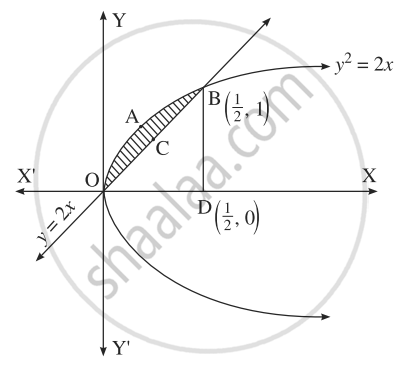Advertisement Remove all ads

# Find the area of the region included between: y2 = 2x and y = 2x - Mathematics and Statistics

Sum

Find the area of the region included between: y2 = 2x and y = 2x

Advertisement Remove all ads

#### Solution

The vertex of the parabola y2 = 2x is at the origin O = (0, 0).To find the points of intersection of the line and the parabola, equaling the values of 2x from both the equations we get,
∴ y2 = y
∴ y2 – y = 0
∴ y(y – 1) = 0
∴ y = 0 or y = 1

When y = 0, x = (0)/(2) = 0

When y = 1, x = (1)/(2)

∴ the points of intersection are O(0, 0) and "B"(1/2, 1)

Required area = area of the region OABCO
= area of the region OABDO – area of the region OCBDO

Now, area of the region OABDO
= area under the parabola y2 = 2x between x = 0 and x = (1)/(2)

= int_0^(1/2)y*dx,  "where"  y = sqrt(2)x

= int_0^(1/2) sqrt(2)xdx

= sqrt(2)[x^(3/2)/(3/2)]_0^(1/2)

= sqrt(2)[2/3 (1/2)^(3/2) - 0]

= sqrt(2)[2/3*1/(2sqrt(2))]

= (1)/(3)
Area of the region OCBDO
= area under the line y
= 2x between x

= 0 and x = (1)/(2)

= int_0^(1/2)y*dx, "where"  y = 2x

= int_0^(1/2)2x*dx

= [(2x^2)/2]_0^(1/2)

= (1)/(4) - 0

= (1)/(4)

∴ required area

= (1)/(3) = (1)/(4)

= (1)/(12) "sq unit".

Concept: Area Bounded by the Curve, Axis and Line
Is there an error in this question or solution?
Advertisement Remove all ads

#### APPEARS IN

Balbharati Mathematics and Statistics 2 (Arts and Science) 12th Standard HSC Maharashtra State Board
Chapter 5 Application of Definite Integration
Exercise 5.1 | Q 3.1 | Page 187
Advertisement Remove all ads
Advertisement Remove all ads
Share
Notifications

View all notifications

Forgot password?